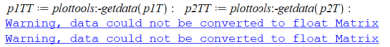## Why Finance:-LatticePrice gives wrong price at 1 &...

I embed Maple code in Mobius for test questions. A simple 2-step CRR tree gives significantly different answer from the calculated by hand or by R's fOptions. Here is the piece of Mobius/Maple:

\$ct = maple("
with(Finance):
S0:=50; X:=50; Ty:=6./12; r:=0.07; sig:=0.3156; n:=1;
dt := Ty/n;
u  := exp(sig*sqrt(dt));
d  := 1/u;
pu := (exp(r*dt) - d)/(u - d);
pd := 1 - pu;
BT := BinomialTree(Ty,n,S0,u,pu,d,pd);
cT := ST -> max(ST - \$X,0);
Ec := EuropeanOption(cT, \$Ty):
LatticePrice(Ec,BT,\$r);
");

The answer is: 6.545478168.  Exactly the same with BlackScholesBinomialTree

The correct answer should be 6.32

In this case the solution by hand is simply e^(-0.07*05)*0.5236*\$12.50

R confirms 6.32:

CRRBinomialTreeOption(TypeFlag = "ce", S=S, X=X, Time=T, r=r, b=b, sigma=sig, n=n)@price

The error decreases with the increase of number of steps. All quantities before LatticePrice are calculated corectly. Any idea of what the reason might be?

Thanks.

## Spin sigma matrices...

Dear,

I would like to do equation of motion calculations using spin matrices.

I would like to do calculations using thecoupling.

Thanks for listening.

## Taking a 2D integral...

I'm trying to use Maple to take the following integral for positive values of a, b, c and non-negative integers i and j.I know that for fixed values of j and k this doable — for example, for j=k=0 Mathematica givesI'm trying the following code in Maple:Am I doing anything wrong?

## a system of pde...

Hi dears

I have a pde system and want to get the solution of this system step by step. is there any command or bunch of commands that could help me?

(pde = partial differential equations)

## Menu bar blurry in maple 2022....

I am using maple 2022 and the menu bar on top (that has restart,execute and other icons) is very blurry.
I tried the instructions here but it did not help.
https://faq.maplesoft.com/s/article/Scaling-issues-when-using-high-resolution-monitors-on-a-Windows-machine?language=en_US

## Why this expression cannot be simplified?...

`simplify(sqrt((x^2 + y^2)/x)/sqrt(x^2 + y^2)) assuming x>0, y>0`

is not sqrt(1/x), why?

## Unable to getdata from a plot error "Warning, data...

Warning, data could not be converted to float Matrix## What is the difference between Physics:-CompactDis...

Hi all,

I am working with the physics package and I want to know if there is a difference between Physics:-CompactDisplay and PDEtools:-declare ?

As well as the difference between Physics:-Simplify and simplify.

For both cases, can they be used as the same ? Are they incompatible in some case?

Thanks !

Kevin

## How to plot Nabla Discrete Mittag - Leffler Functi...

How to plot Nabla Discrete Mittag - Leffler Function in Maple?

## possible transformations...

How can we unveil all the possible transformations that reduce the number of independent variables of a given pde? I tried it by using "InvarientSolutions" which gives eight possible transformations. But under those transformations, I got only two different odes. Is there any other method/command from which we can get other possible odes of a given pde?

pde_ode.mw

## How i solve highly non-linear coupled differential...The transformed boundary conditions are